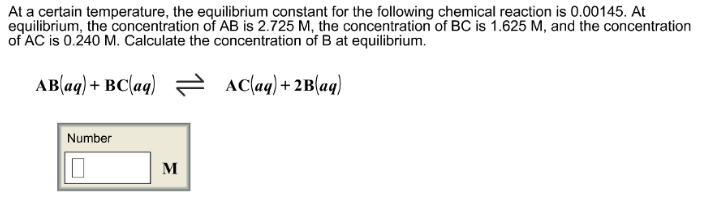# Problem: At a certain temperature, the equilibrium constant for the following chemical reaction is 0.00145. At equilibrium, the concentration of AB is 2.725 M, the concentration of BC is 1.625 M, and the concentration of AC is 0.240 M. Calculate the concentration of B at equilibrium. AB (aq) + BC (aq) ⇌ AC (aq) + 2B (aq)

###### FREE Expert Solution
79% (307 ratings)###### Problem Details

At a certain temperature, the equilibrium constant for the following chemical reaction is 0.00145. At equilibrium, the concentration of AB is 2.725 M, the concentration of BC is 1.625 M, and the concentration of AC is 0.240 M. Calculate the concentration of B at equilibrium.

AB (aq) + BC (aq) ⇌ AC (aq) + 2B (aq)